# History

27 de Jul de 2015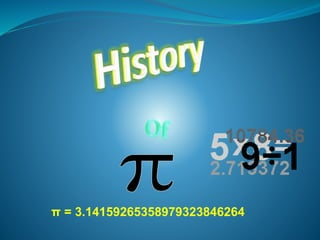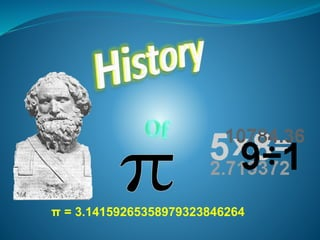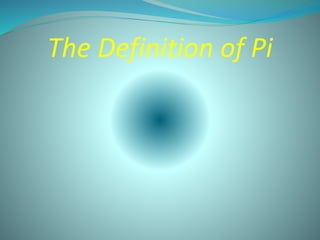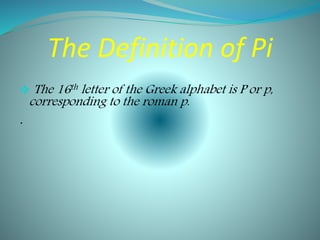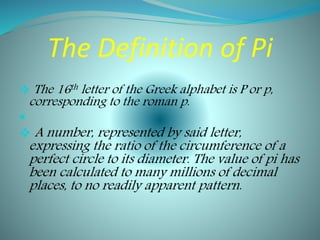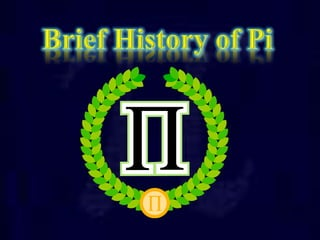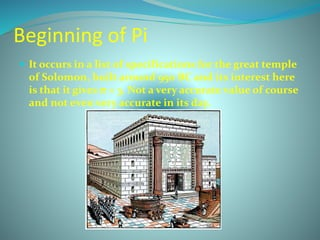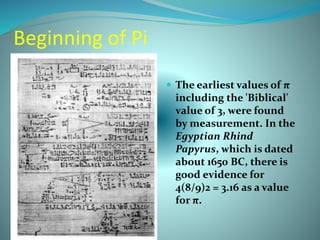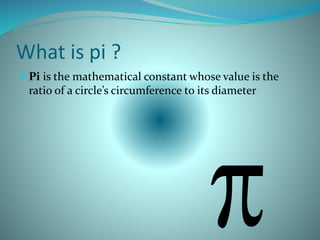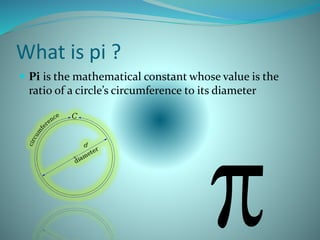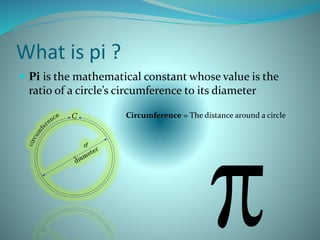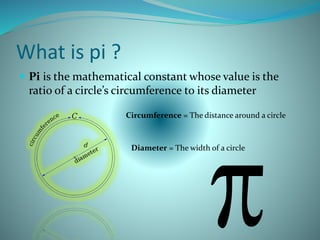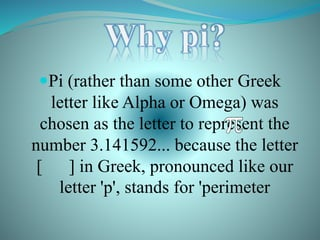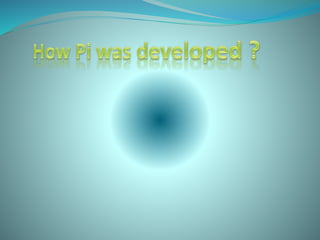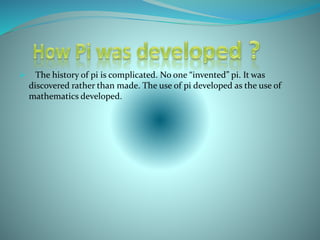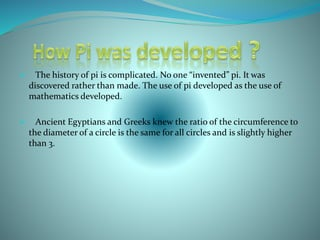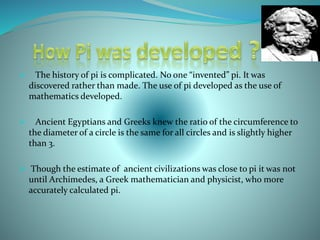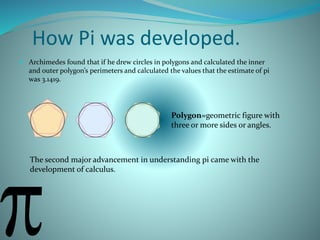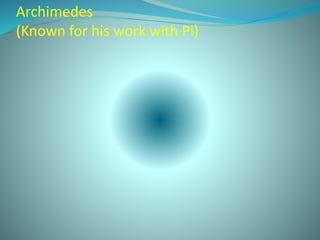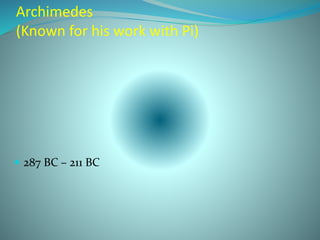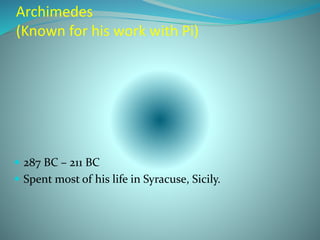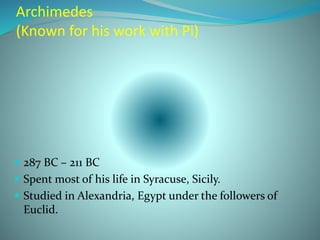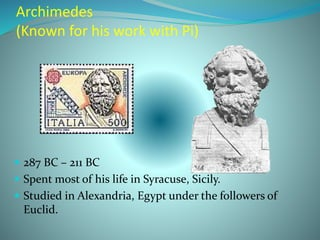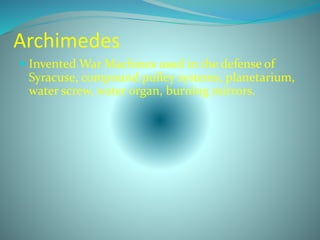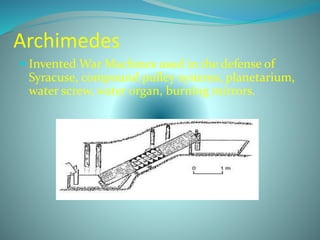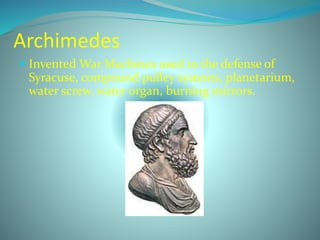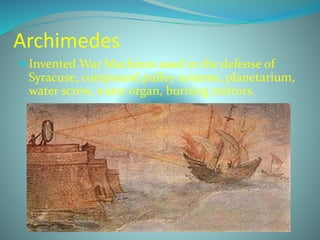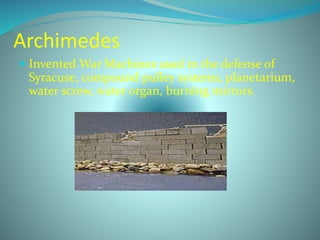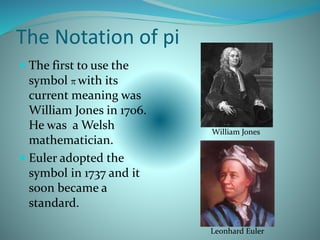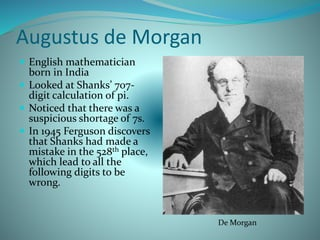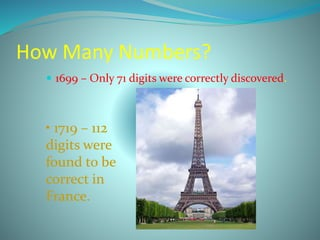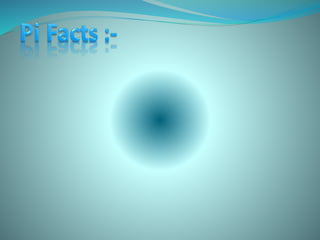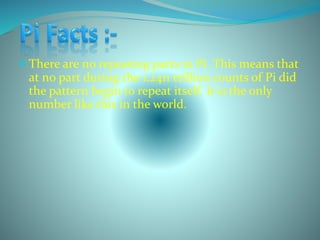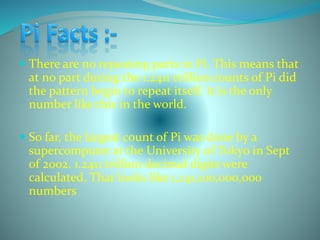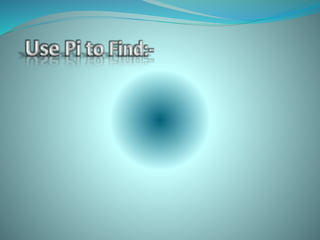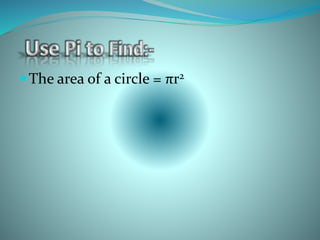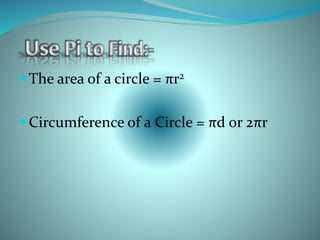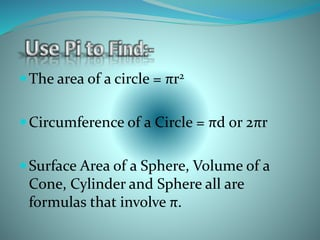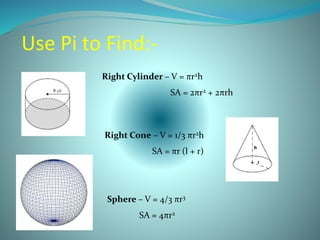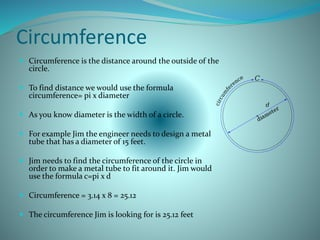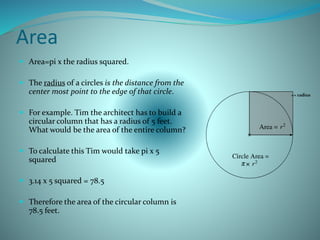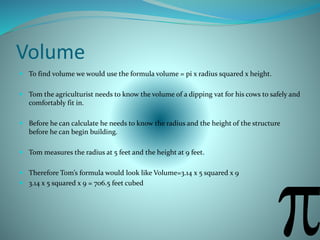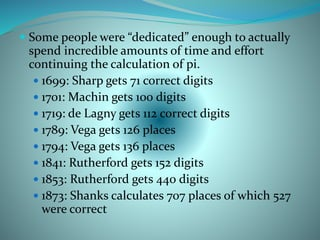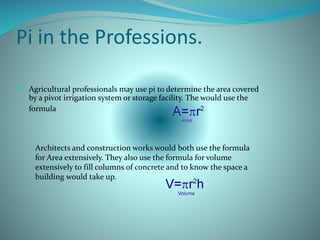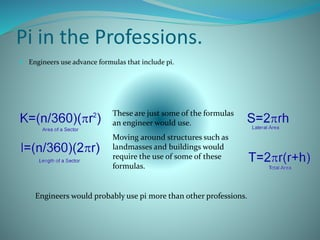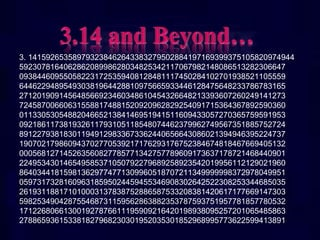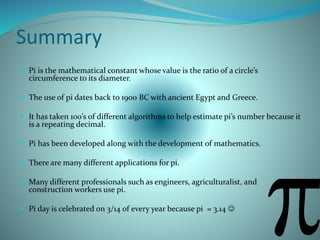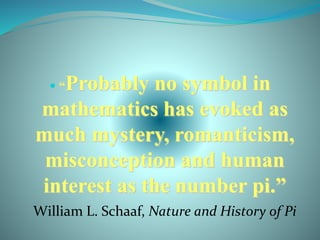1 de 51

### History

• 5. The Definition of Pi  The 16th letter of the Greek alphabet is P or p, corresponding to the roman p. .
• 6. The Definition of Pi  The 16th letter of the Greek alphabet is P or p, corresponding to the roman p.   A number, represented by said letter, expressing the ratio of the circumference of a perfect circle to its diameter. The value of pi has been calculated to many millions of decimal places, to no readily apparent pattern.
• 8. Beginning of Pi  It occurs in a list of specifications for the great temple of Solomon, built around 950 BC and its interest here is that it gives π = 3. Not a very accurate value of course and not even very accurate in its day.
• 9. Beginning of Pi  The earliest values of π including the 'Biblical' value of 3, were found by measurement. In the Egyptian Rhind Papyrus, which is dated about 1650 BC, there is good evidence for 4(8/9)2 = 3.16 as a value for π.
• 10. What is pi ?  Pi is the mathematical constant whose value is the ratio of a circle’s circumference to its diameter
• 11. What is pi ?  Pi is the mathematical constant whose value is the ratio of a circle’s circumference to its diameter
• 12. What is pi ?  Pi is the mathematical constant whose value is the ratio of a circle’s circumference to its diameter Circumference = The distance around a circle
• 13. What is pi ?  Pi is the mathematical constant whose value is the ratio of a circle’s circumference to its diameter Circumference = The distance around a circle Diameter = The width of a circle
• 14. Pi (rather than some other Greek letter like Alpha or Omega) was chosen as the letter to represent the number 3.141592... because the letter [ ] in Greek, pronounced like our letter 'p', stands for 'perimeter
• 16.  The history of pi is complicated. No one “invented” pi. It was discovered rather than made. The use of pi developed as the use of mathematics developed.
• 17.  The history of pi is complicated. No one “invented” pi. It was discovered rather than made. The use of pi developed as the use of mathematics developed.  Ancient Egyptians and Greeks knew the ratio of the circumference to the diameter of a circle is the same for all circles and is slightly higher than 3.
• 18.  The history of pi is complicated. No one “invented” pi. It was discovered rather than made. The use of pi developed as the use of mathematics developed.  Ancient Egyptians and Greeks knew the ratio of the circumference to the diameter of a circle is the same for all circles and is slightly higher than 3.  Though the estimate of ancient civilizations was close to pi it was not until Archimedes, a Greek mathematician and physicist, who more accurately calculated pi.
• 19. How Pi was developed.  Archimedes found that if he drew circles in polygons and calculated the inner and outer polygon’s perimeters and calculated the values that the estimate of pi was 3.1419. The second major advancement in understanding pi came with the development of calculus. Polygon=geometric figure with three or more sides or angles.
• 20. Archimedes (Known for his work with Pi)
• 21. Archimedes (Known for his work with Pi)  287 BC – 211 BC
• 22. Archimedes (Known for his work with Pi)  287 BC – 211 BC  Spent most of his life in Syracuse, Sicily.
• 23. Archimedes (Known for his work with Pi)  287 BC – 211 BC  Spent most of his life in Syracuse, Sicily.  Studied in Alexandria, Egypt under the followers of Euclid.
• 24. Archimedes (Known for his work with Pi)  287 BC – 211 BC  Spent most of his life in Syracuse, Sicily.  Studied in Alexandria, Egypt under the followers of Euclid.
• 25. Archimedes  Invented War Machines used in the defense of Syracuse, compound pulley systems, planetarium, water screw, water organ, burning mirrors.
• 26. Archimedes  Invented War Machines used in the defense of Syracuse, compound pulley systems, planetarium, water screw, water organ, burning mirrors.
• 27. Archimedes  Invented War Machines used in the defense of Syracuse, compound pulley systems, planetarium, water screw, water organ, burning mirrors.
• 28. Archimedes  Invented War Machines used in the defense of Syracuse, compound pulley systems, planetarium, water screw, water organ, burning mirrors.
• 29. Archimedes  Invented War Machines used in the defense of Syracuse, compound pulley systems, planetarium, water screw, water organ, burning mirrors.
• 30. The Notation of pi  The first to use the symbol π with its current meaning was William Jones in 1706. He was a Welsh mathematician.  Euler adopted the symbol in 1737 and it soon became a standard. William Jones Leonhard Euler
• 31. Augustus de Morgan  English mathematician born in India  Looked at Shanks’ 707- digit calculation of pi.  Noticed that there was a suspicious shortage of 7s.  In 1945 Ferguson discovers that Shanks had made a mistake in the 528th place, which lead to all the following digits to be wrong. De Morgan
• 32. How Many Numbers?  1699 – Only 71 digits were correctly discovered. • 1719 – 112 digits were found to be correct in France.
• 34.  There are no repeating parts in Pi. This means that at no part during the 1.2411 trillion counts of Pi did the pattern begin to repeat itself. It is the only number like this in the world.
• 35.  There are no repeating parts in Pi. This means that at no part during the 1.2411 trillion counts of Pi did the pattern begin to repeat itself. It is the only number like this in the world.  So far, the largest count of Pi was done by a supercomputer at the University of Tokyo in Sept of 2002. 1.2411 trillion decimal digits were calculated. That looks like 1,241,100,000,000 numbers
• 37. The area of a circle = πr2
• 38. The area of a circle = πr2 Circumference of a Circle = πd or 2πr
• 39. The area of a circle = πr2 Circumference of a Circle = πd or 2πr Surface Area of a Sphere, Volume of a Cone, Cylinder and Sphere all are formulas that involve π.
• 40. Use Pi to Find:- Right Cylinder – V = πr2h SA = 2πr2 + 2πrh Right Cone – V = 1/3 πr2h SA = πr (l + r) Sphere – V = 4/3 πr3 SA = 4πr2
• 41. Circumference  Circumference is the distance around the outside of the circle.  To find distance we would use the formula circumference= pi x diameter  As you know diameter is the width of a circle.  For example Jim the engineer needs to design a metal tube that has a diameter of 15 feet.  Jim needs to find the circumference of the circle in order to make a metal tube to fit around it. Jim would use the formula c=pi x d  Circumference = 3.14 x 8 = 25.12  The circumference Jim is looking for is 25.12 feet.
• 42. Area  Area=pi x the radius squared.  The radius of a circles is the distance from the center most point to the edge of that circle.  For example. Tim the architect has to build a circular column that has a radius of 5 feet. What would be the area of the entire column?  To calculate this Tim would take pi x 5 squared  3.14 x 5 squared = 78.5  Therefore the area of the circular column is 78.5 feet. <= radius
• 43. Volume  To find volume we would use the formula volume = pi x radius squared x height.  Tom the agriculturist needs to know the volume of a dipping vat for his cows to safely and comfortably fit in.  Before he can calculate he needs to know the radius and the height of the structure before he can begin building.  Tom measures the radius at 5 feet and the height at 9 feet.  Therefore Tom’s formula would look like Volume=3.14 x 5 squared x 9  3.14 x 5 squared x 9 = 706.5 feet cubed
• 44.  Some people were “dedicated” enough to actually spend incredible amounts of time and effort continuing the calculation of pi.  1699: Sharp gets 71 correct digits  1701: Machin gets 100 digits  1719: de Lagny gets 112 correct digits  1789: Vega gets 126 places  1794: Vega gets 136 places  1841: Rutherford gets 152 digits  1853: Rutherford gets 440 digits  1873: Shanks calculates 707 places of which 527 were correct
• 45. Pi in the Professions.  Agricultural professionals may use pi to determine the area covered by a pivot irrigation system or storage facility. The would use the formula Architects and construction works would both use the formula for Area extensively. They also use the formula for volume extensively to fill columns of concrete and to know the space a building would take up.
• 46. Pi in the Professions.  Engineers use advance formulas that include pi. These are just some of the formulas an engineer would use. Moving around structures such as landmasses and buildings would require the use of some of these formulas. Engineers would probably use pi more than other professions.
• 47. 3. 141592653589793238462643383279502884197169399375105820974944 592307816406286208998628034825342117067982148086513282306647 093844609550582231725359408128481117450284102701938521105559 644622948954930381964428810975665933446128475648233786783165 271201909145648566923460348610454326648213393607260249141273 724587006606315588174881520920962829254091715364367892590360 011330530548820466521384146951941511609433057270365759591953 092186117381932611793105118548074462379962749567351885752724 891227938183011949129833673362440656643086021394946395224737 190702179860943702770539217176293176752384674818467669405132 000568127145263560827785771342757789609173637178721468440901 224953430146549585371050792279689258923542019956112129021960 864034418159813629774771309960518707211349999998372978049951 059731732816096318595024459455346908302642522308253344685035 261931188171010003137838752886587533208381420617177669147303 598253490428755468731159562863882353787593751957781857780532 171226806613001927876611195909216420198938095257201065485863 278865936153381827968230301952035301852968995773622599413891
• 48. Summary  Pi is the mathematical constant whose value is the ratio of a circle’s circumference to its diameter.  The use of pi dates back to 1900 BC with ancient Egypt and Greece.  It has taken 100’s of different algorithms to help estimate pi’s number because it is a repeating decimal.  Pi has been developed along with the development of mathematics.  There are many different applications for pi.  Many different professionals such as engineers, agriculturalist, and construction workers use pi.  Pi day is celebrated on 3/14 of every year because pi = 3.14 
• 49.  “Probably no symbol in mathematics has evoked as much mystery, romanticism, misconception and human interest as the number pi.” William L. Schaaf, Nature and History of Pi